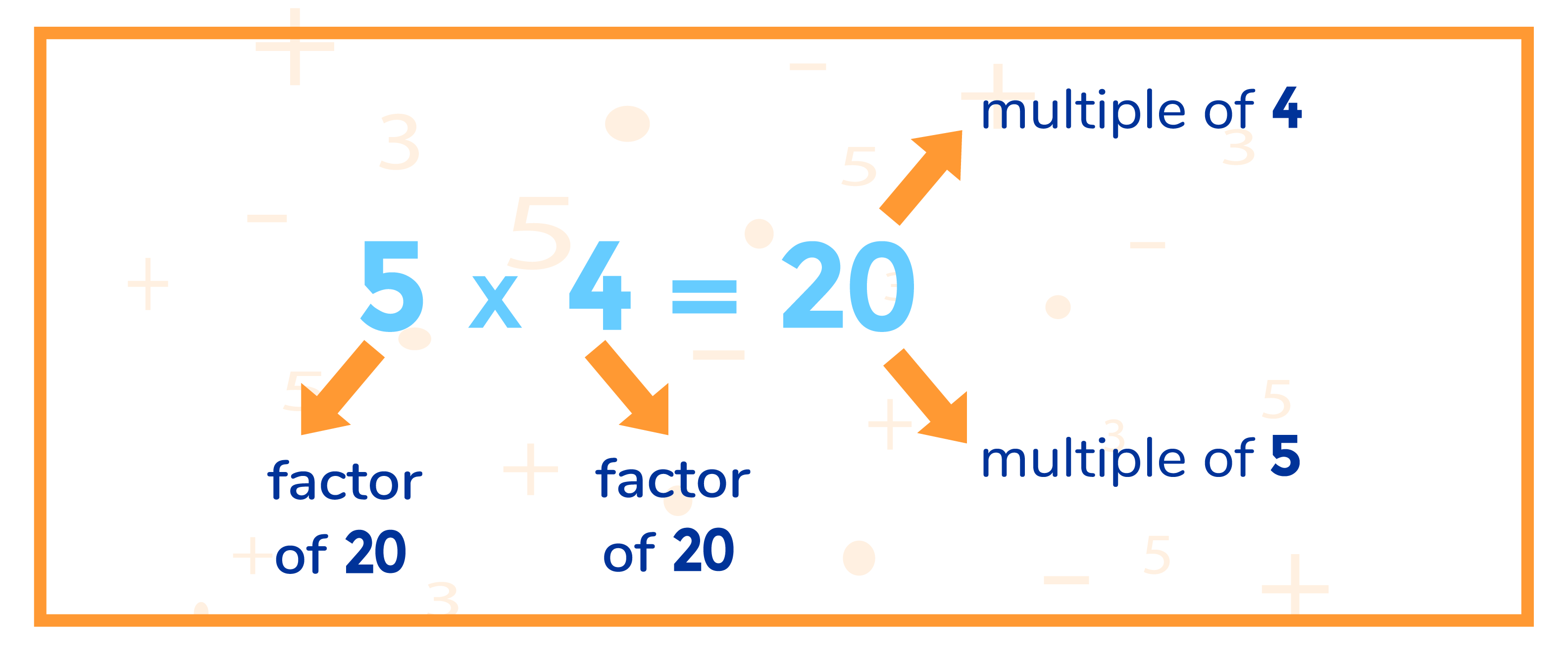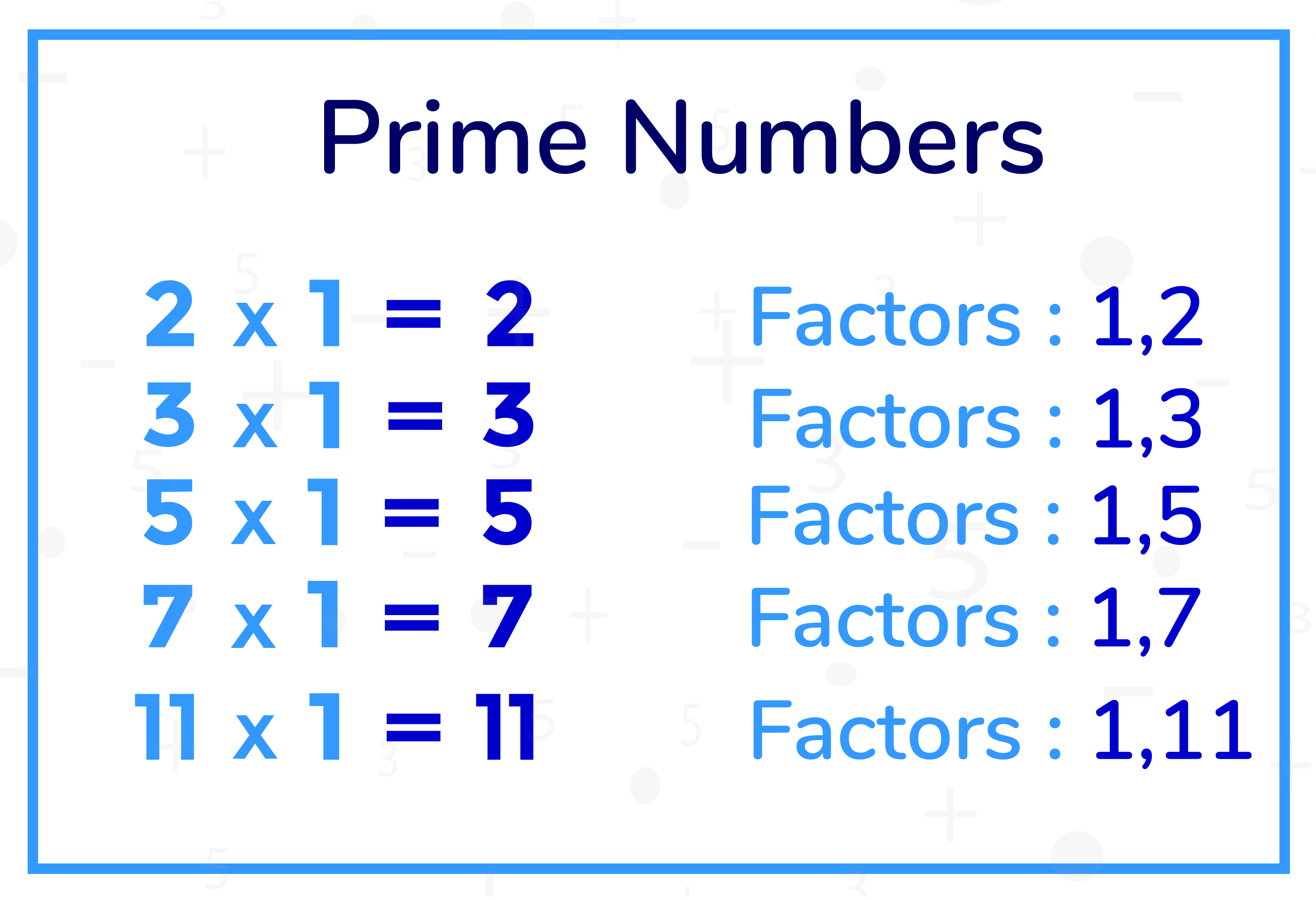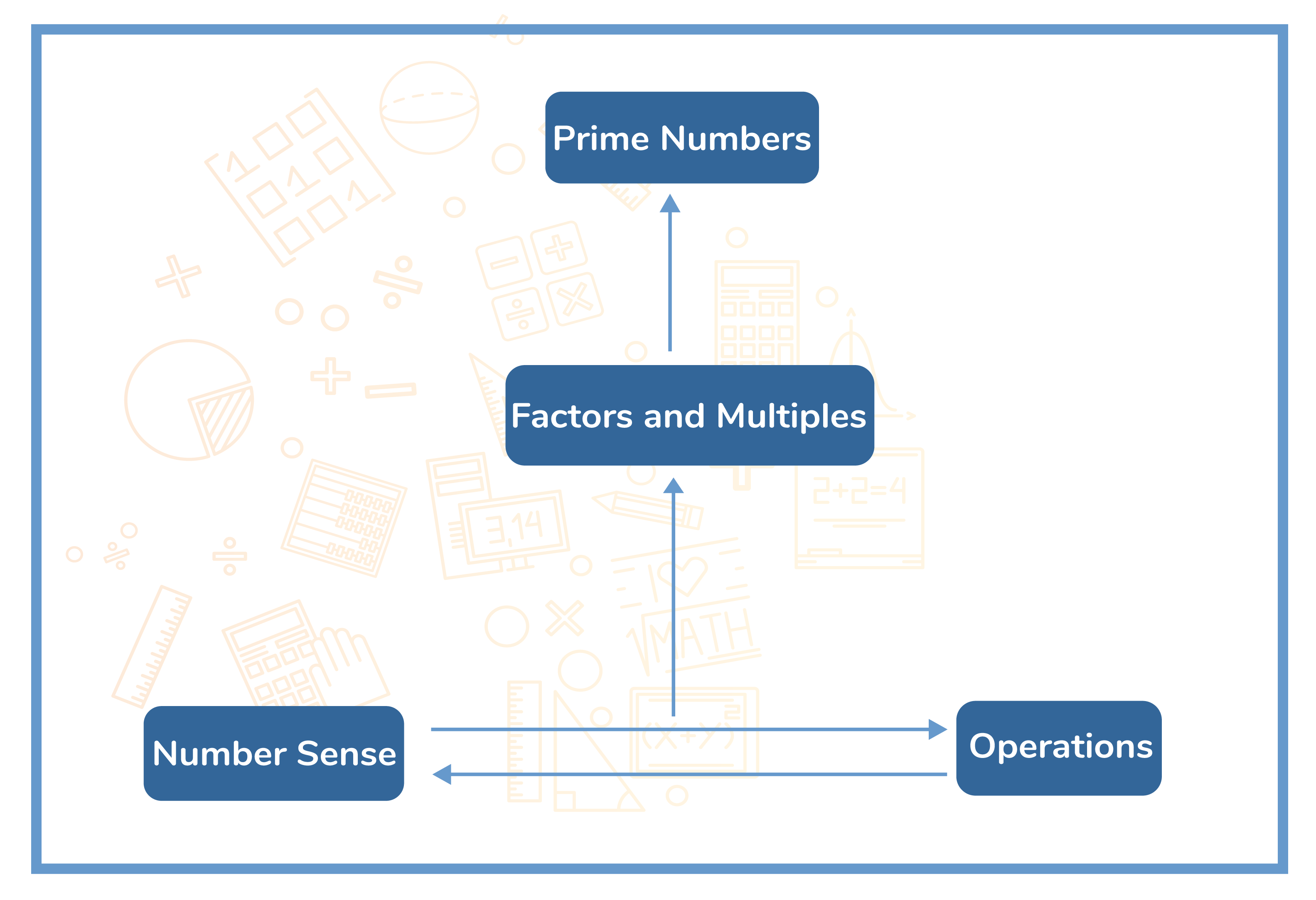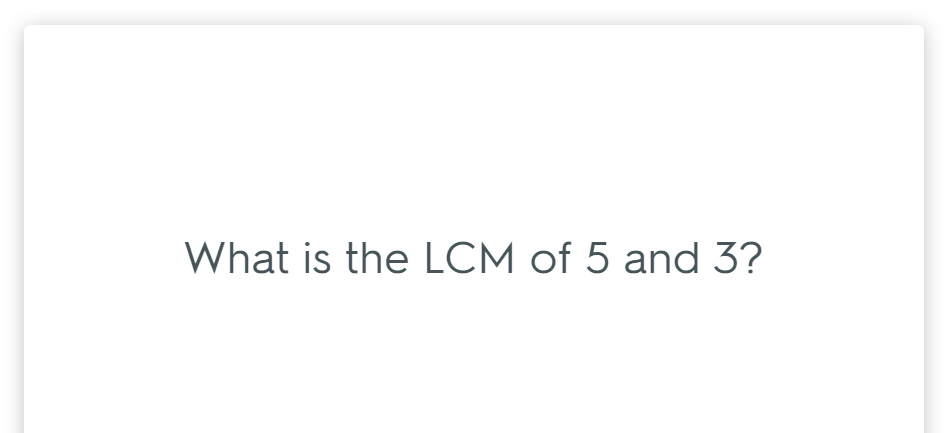In the verge of coronavirus pandemic, we are providing FREE access to our entire Online Curriculum to ensure Learning Doesn't STOP!

# Factors, Multiples and Prime Numbers

Go back to  'Maths'

## Factors, Multiples and Prime Numbers

We have so far seen how to recognize and conduct the basic operations of addition, subtraction, multiplication and division using numbers. These operations are merely the beginning. Mastery of these simply pave the way to more advanced, complicated and powerful operations along with one of the most interesting group of numbers. Factors and multiples represent an evolution in the application of basic operations. They help in carrying out more advanced calculations in an easier and more succinct manner.

### What are Factors?

Any number that divides another number leaving a remainder of zero is called its Factor.

### What are Multiples?

Any number that features in the times table of a particular number is called its Multiple.### When a number only has two factors, one and itself is called a Prime Number.## Topics closely related to Factors, multiples and Primes

The image given below shows how Factors, Multiples and Prime Numbers are connected to other topics. To understand Factors and Multiples one should be proficient at topics like multiplication, division and divisibility tests. Must have a good number sense, a good grasp on operations with numbers.## Sub Topics

Topics in Math are interdependent. Studying them in a sequence will lead to a better understanding. A Cuemath student learns all about Factor, Multiples and Primes in the following sequence.

## Simple ways to learn Factors, Multiples and Prime Numbers

Like any other topic in arithmetic, the key to proper understanding is a strong grasp of the basics and practice. The most effective ways to ensure that your child excels with Factors, Multiples and Prime Numbers are:

Flash Cards: These are an excellent way of keeping concepts at your fingertips. Dedicate thirty minutes of your child’s study routine to make them go through the concepts of factors, multiples and prime numbers. This exercise not only strengthens core concepts but also build mental math abilities.## FAQ

Q1. Can prime numbers be multiples?

No, prime numbers cannot be multiples. Multiples of a number are numbers found by multiplying the original number by a whole number whereas prime numbers are the numbers with only two distinct factors. For example, let’s take $$2$$ as the prime number as it is divisible by $$1$$ and itself but $$2$$ can have various infinite multiples when multiplied by the whole numbers.

Q2. What is the difference between prime factors & prime numbers?

Prime factors are any of the prime numbers that can be multiplied to give the number. Example: The prime factors of $$15$$ are $$3$$ and $$5$$ (because $$3 \times 5 = 15,$$ and $$3$$ and $$5$$ are prime numbers) whereas Prime numbers are numbers that have exactly two factors $$1$$ and itself.

Q3. What is the difference between multiple & factor?

A factor is a number that leaves no remainder behind after it divides the number. On the contrary, multiple is a number reached by multiplying a given number by another. While factors of a number are finite, multiples are infinite.

Q4. Is $$0$$ a prime number?

0 is not a prime number. Prime Numbers are numbers greater than $$1,$$ because Prime Numbers should have $$1$$ and the number itself, as its factor, which means, it must have only two positive factors. Also, zero has an infinite number of divisors (any nonzero whole number divides zero).

Q5. Why $$1$$ is not a prime number?

$$1$$ is NOT a prime number because it does not satisfy the definition of a prime number.

Q6. Prime numbers between $$1$$ & $$100?$$

$$2, 3, 5, 7, 11, 13, 17, 19, 23, 29, 31, 37, 41, 43, 47, 53, 59, 61, 67, 71, 73, 79, 83, 89,$$ and $$97.$$